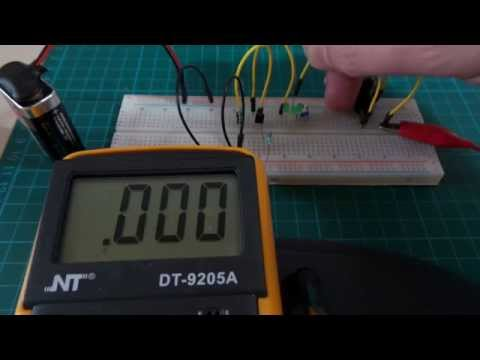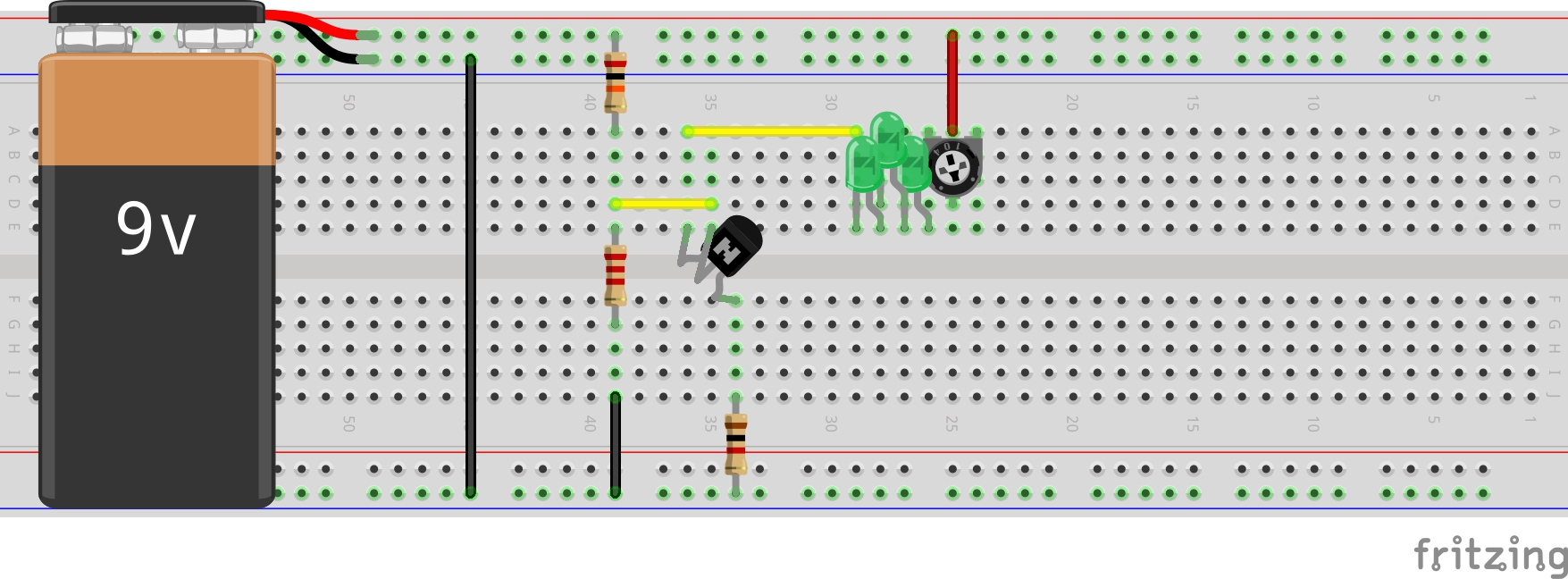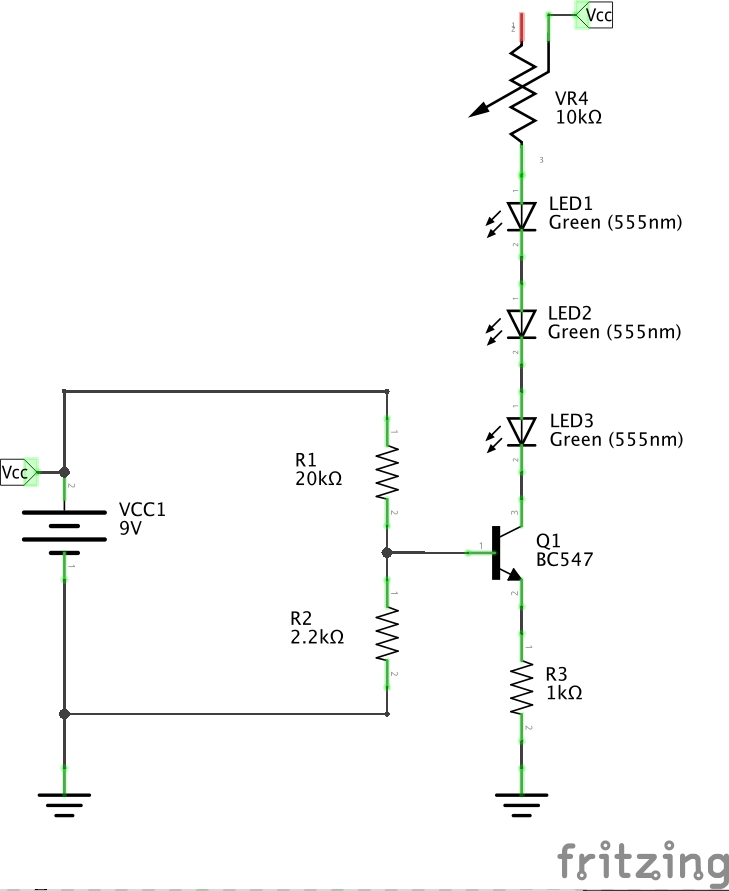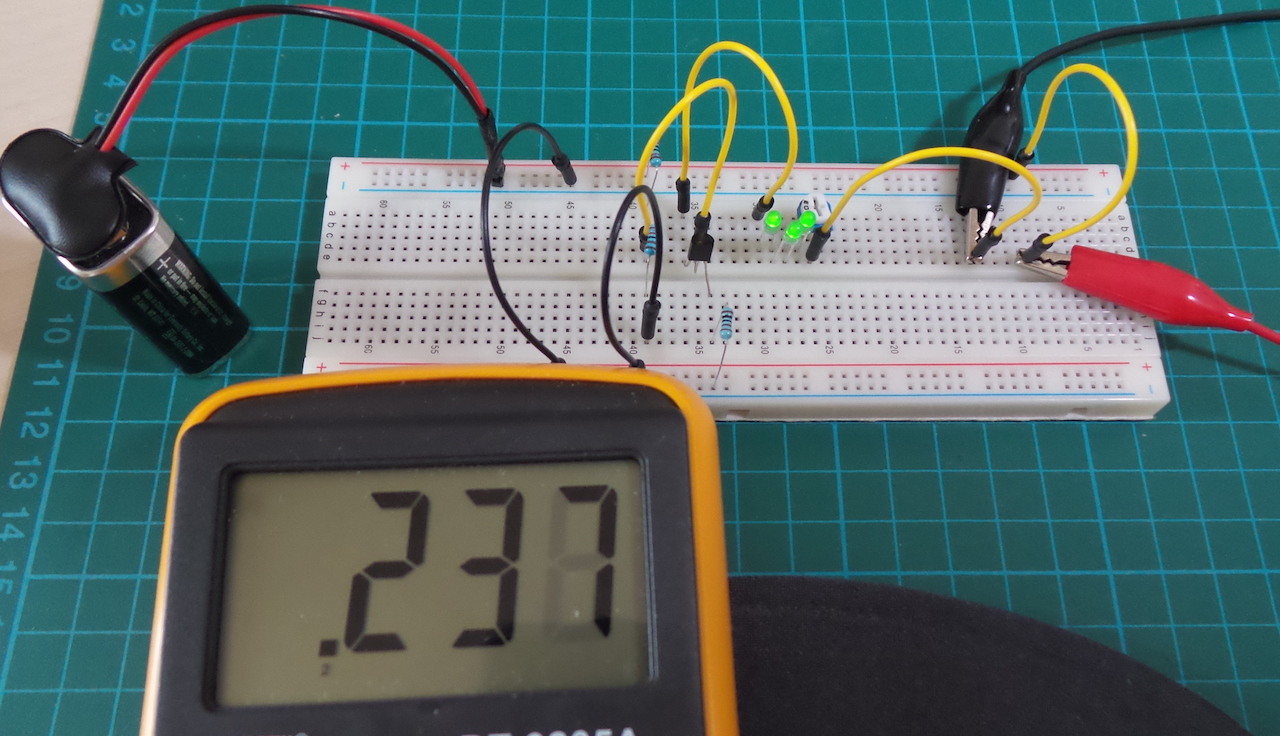# #093 BJT CurrentSink

Test a BJT constant current sink circuit.

Here’s a quick demo of the circuit in action:Current sources and sinks are excellently described in w2aew’s tutorial on YouTube.

This circuit uses a voltage divider bias for a BC547 NPN transistor. Assuming the current through the voltage divider is much greater than the base current i.e. I(R1) » Ib then Vb is approx 0.892V

From the BC547 datasheet, Vbe ranges from 0.55 (on) to 0.7V (saturated), so let’s take 0.6V for design purposes. So Ve = Vb - Vbe = 0.29V

Given this bias, the emitter current is determined by the value of resistor R3. And since we assume Ib is negligible, the collector current is the same. With a 1kΩ resistor, Ie = Ve/R3 = 290μA.

Actually my battery is running low and only delivering 8.41V so I’m expecting my constant current to be around: Ic = Ie = 230μA

In the test circuit, I’ve added a load of 3 green LEDS (with a forward voltage of about 1.9-2V) and a 10kΩ trimmer pot VR4.

When measure in circuit, the current is stable at 239μA as expected. This remain’s stable, even as the load changes by varying resistance VR4 or reducing the number of LEDs.

## Construction